# Thin, Thick Cylinders & Spheres Notes | Study Mechanical Engineering SSC JE (Technical) - Mechanical Engineering

## Mechanical Engineering: Thin, Thick Cylinders & Spheres Notes | Study Mechanical Engineering SSC JE (Technical) - Mechanical Engineering

The document Thin, Thick Cylinders & Spheres Notes | Study Mechanical Engineering SSC JE (Technical) - Mechanical Engineering is a part of the Mechanical Engineering Course Mechanical Engineering SSC JE (Technical).
All you need of Mechanical Engineering at this link: Mechanical Engineering

THIN & THICK CYLINDERS AND SPHERES

THIN CYLINDERS

•  If the thickness of the cylinder is less than 1/10 to 1/15 of the diameter of the cylinder,  it is treated as the thin cylinder.
•  It is assumed that the stresses are uniformly distributed through out the thickness ofthe wall.
•  'Hoop stress’ or ‘Circumferential stress’ is given by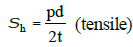•   ‘Longitudinal stress’ is given by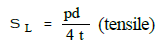•  The Maximum shear stress is given by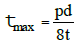•  Hoop strain is given by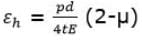•  Longitudinal strain is given by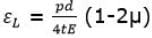•  Volumetric strain is given by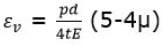Where
p = internal pressure
d = diameter of cylinder
t = thickness of the cylinder
μ = Poisson's ratio

•  If ‘sa’ be the permissible tensile stress for the shell material, then from strength  point of view, the major principal stress (s) should be less than or equal to sa

Hence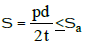or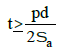THIN SPHERICAL SHELLS

•  In case of thin spherical shells, longitudinal stress and circumferential stress are equal and are given by

s1 =s2 =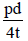(tensile in nature)

•  The maximum shear stress,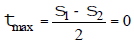•  The strain in any direction is given by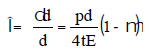•   The volumetric strain is given by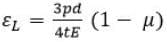CYLINDERS WITH HEMISPHERICAL ENDS

Let tc = thickness of the cylinder
ts = thickness of the hemisphere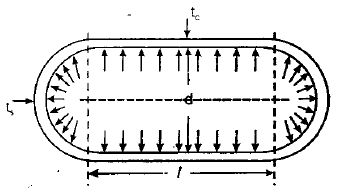•   Hoop stress in cylindrical part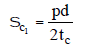•   Hoop stress in hemispherical part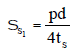•  Longitudinal stress in cylindrical part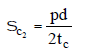•  Longitudinal stress in hemispherical part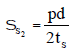•   Circumferential strain in hemispherical part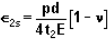•   Circumferential strain in cylindrical part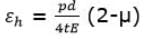•   For the condition of no distortion of the junction

Thus, equating the two strains in order that there shall be no distortion of the junction.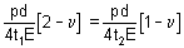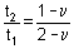This means thickness of cylindrical part should be more than the hemispherical part.

•  For the condition of same maximum stress in cylindrical and hemispherical parts,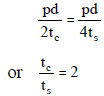THICK CYLINDRICAL SHELL

•   If the thickness of shell is greater than of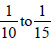its diameter then it is called thick shell.

•   In thick cylinders the circumferential stress no longer remains constant, but varies  along the thickness and the radial pressure (px) is also not negligible.

The following three types of stresses are existing in thick cylinders :

(i) The radial pressure ‘px’ (compressive)
(ii) The hoop stress fx (tensile)
(iii) The longitudinal tensile stress po (tensile)

•  The longitudinal stress may be given by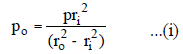Hoop stress, is given by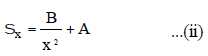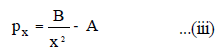Equation (ii) and (iii) are called Lame’s equation.
ro = outer radius of shell
ri = inner radius of shell
A and B are Lame’s constant

Note:-

1. Longitudinal tension is uniform across the thickness.
2. Hoop tension vary form maximum at inner face to minimum at outer face hyperbolically.
3. Radial compression varies from maximum at inner face to zero at outer face (atm.) hyperbolically.

The document Thin, Thick Cylinders & Spheres Notes | Study Mechanical Engineering SSC JE (Technical) - Mechanical Engineering is a part of the Mechanical Engineering Course Mechanical Engineering SSC JE (Technical).
All you need of Mechanical Engineering at this link: Mechanical EngineeringUse Code STAYHOME200 and get INR 200 additional OFF

## Mechanical Engineering SSC JE (Technical)

6 videos|89 docs|55 tests

Track your progress, build streaks, highlight & save important lessons and more!

,

,

,

,

,

,

,

,

,

,

,

,

,

,

,

,

,

,

,

,

,

,

,

,

;